# Generative Adversarial Networks, an overview

In this article, we’ll explain GANs by applying them to the task of generating images. One of the few successful techniques in unsupervised machine learning, and are quickly revolutionizing our ability to perform generative tasks.comments

By Keshav Dhandhania, Co-Founder, Compose Labs & Arash Delijani, Co-Founder, Orderly.

In this article, I’ll talk about Generative Adversarial Networks, or GANs for short. GANs are one of the very few machine learning techniques which has given good performance for generative tasks, or more broadly unsupervised learning. In particular, they have given splendid performance for a variety of image generation related tasks. Yann LeCun, one of the forefathers of deep learning, has called them “the best idea in machine learning in the last 10 years”. Most importantly, the core conceptual ideas associated with a GAN are quite simple to understand (and in-fact, you should have a good idea about them by the time you finish reading this article).

In this article, we’ll explain GANs by applying them to the task of generating images. The following is the outline of this article

1. A brief review of Deep Learning
2. The image generation problem
3. Key issue in generative tasks
4. Generative Adversarial Networks
5. Challenges
7. Conclusion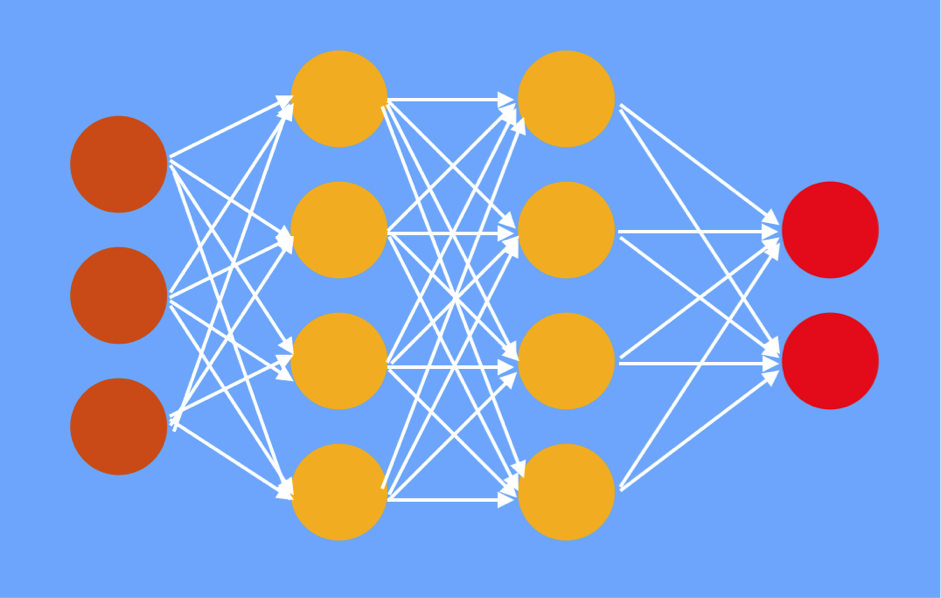Sketch of a (feed-forward) neural network, with input layer in brown, hidden layers in yellow, and output layer in red.

A brief review of Deep Learning

Let’s begin with a brief overview of deep learning. Above, we have a sketch of a neural network. The neural network is made of up neurons, which are connected to each other using edges. The neurons are organized into layers – we have the hidden layers in the middle, and the input and output layers on the left and right respectively. Each of the edges is weighted, and each neuron performs a weighted sum of values from neurons connected to it by incoming edges, and thereafter applies a nonlinear activation such as sigmoid or ReLU. For example, neurons in the first hidden layer, calculate a weighted sum of neurons in the input layer, and then apply the ReLU function. The activation function introduces a nonlinearity which allows the neural network to model complex phenomena (multiple linear layers would be equivalent to a single linear layer).

Given a particular input, we sequentially compute the values outputted by each of the neurons (also called the neurons’ activity). We compute the values layer by layer, going from left to right, using already computed values from the previous layers. This gives us the values for the output layer. Then we define a cost, based on the values in the output layer and the desired output (target value). For example, a possible cost function is the mean-squared error cost function.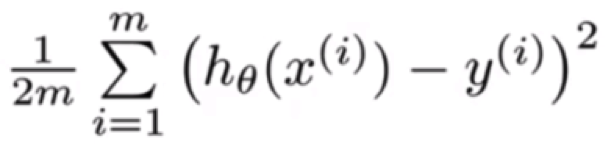where, x is the input, h(x) is the output and y is the target. The sum is over the various data points in our dataset.

At each step, our goal is to nudge each of the edge weights by the right amount so as to reduce the cost function as much as possible. We calculate a gradient, which tells us how much to nudge each weight. Once we compute the cost, we compute the gradients using the backpropagation algorithm. The main result of the backpropagation algorithm is that we can exploit the chain rule of differentiation to calculate the gradients of a layer given the gradients of the weights in layer above it. Hence, we calculate these gradients backwards, i.e. from the output layer to the input layer. Then, we update each of the weights by an amount proportional to the respective gradients (i.e. gradient descent).

If you would like to read about neural networks and the back-propagation algorithm in more detail, I recommend reading this article by Nikhil Buduma on Deep Learning in a Nutshell.

The image generation problem

In the image generation problem, we want the machine learning model to generate images. For training, we are given a dataset of images (say 1,000,000 images downloaded from the web). During testing, the model should generate images that look like they belong to the training dataset, but are not actually in the training dataset. That is, we want to generate novel images (in contrast to simply memorizing), but we still want it to capture patterns in the training dataset so that new images feel like they look similar to those in the training dataset.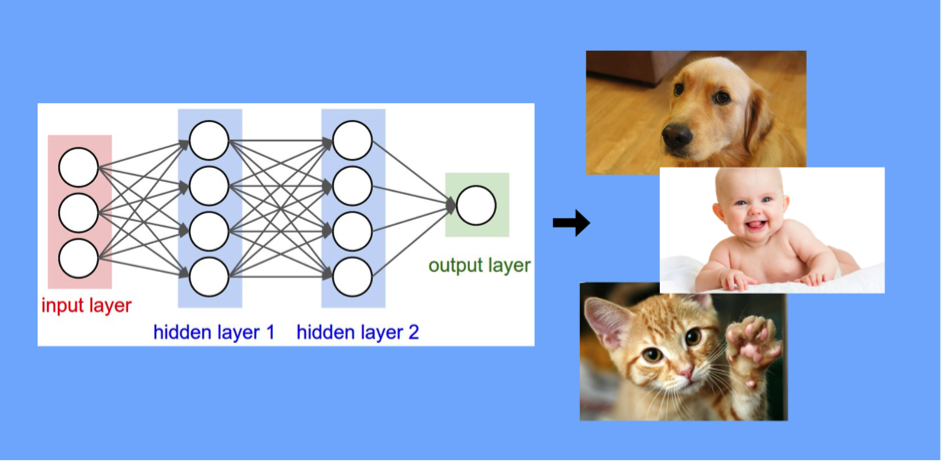Image generation problem: There is no input, and the desired output is an image.

One thing to note: there is no input in this problem during the testing or prediction phase. Everytime we ‘run the model’, we want it to generate (output) a new image. This can be achieved by saying that the input is going to be sampled randomly from a distribution that is easy to sample from (say the uniform distribution or Gaussian distribution).

Key issue in generative tasks

The crucial issue in a generative task is – what is a good cost function? Let’s say you have two images that are outputted by a machine learning model. How do we decide which one is better, and by how much?

The most common solution to this question in previous approaches has been, distance between the output and its closest neighbor in the training dataset, where the distance is calculated using some predefined distance metric. For example, in the language translation task, we usually have one source sentence, and a small set of (about 5) target sentences, i.e. translations provided by different human translators. When a model generates a translation, we compare the translation to each of the provided targets, and assign it the score based on the target it is closest to (in particular, we use the BLEU score, which is a distance metric based on how many n-grams match between the two sentences). That kind of works for single sentence translations, but the same approach leads to a significant deterioration in the quality of the cost function when the target is a larger piece of text. For example, our task could be to generate a paragraph length summary of a given article. This deterioration stems from the inability of the small number of samples to represent the wide range of variation observed in all possible correct answers.

GANs answer to the above question is, use another neural network! This scorer neural network (called the discriminator) will score how realistic the image outputted by the generator neural network is. These two neural networks have opposing objectives (hence, the word adversarial). The generator network’s objective is to generate fake images that look real, the discriminator network’s objective is to tell apart fake images from real ones.

This puts generative tasks in a setting similar to the 2-player games in reinforcement learning (such as those of chess, Atari games or Go) where we have a machine learning model improving continuously by playing against itself, starting from scratch. The difference here is that often in games like chess or Go, the roles of the two players are symmetric (although not always). For GAN setting, the objectives and roles of the two networks are different, one generates fake samples, the other distinguishes real ones from fake ones.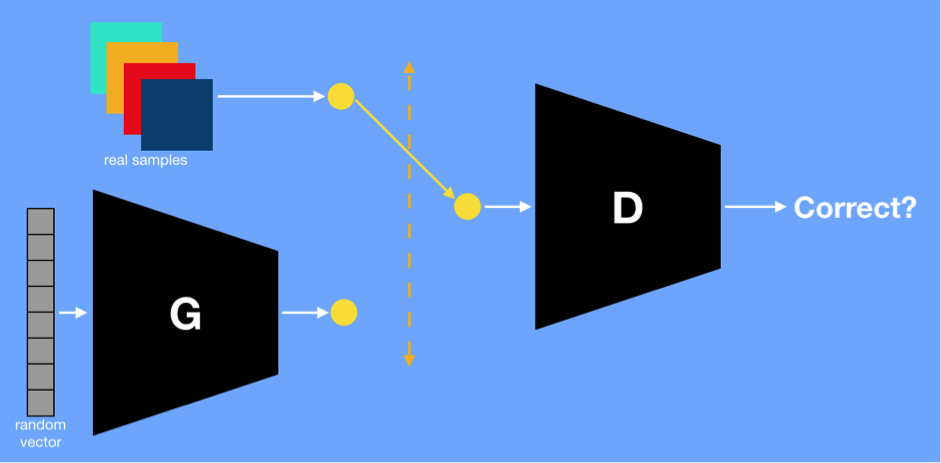Sketch of Generative Adversarial Network, with the generator network labelled as G and the discriminator network labelled as D

Above, we have a diagram of a Generative Adversarial Network. The generator network G and discriminator network D are playing a 2-player minimax game. First, to better understand the setup, notice that D’s inputs can be sampled from the training data or the output generated by G: Half the time from one and half the time from the other. To generate samples from G, we sample the latent vector from the Gaussian distribution and then pass it through G. If we are generating a 200 x 200 grayscale image, then G’s output is a 200 x 200 matrix. The objective function is given by the following function, which is essentially the standard log-likelihood for the predictions made by D: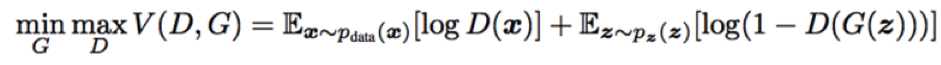The generator network G is minimizing the objective, i.e. reducing the log-likelihood, or trying to confuse D. It wants D to identify the the inputs it receives from G as correct whenever samples are drawn from its output. The discriminator network D is maximizing the objective, i.e. increasing the log-likelihood, or trying to distinguish generated samples from real samples. In other words, if G does a good job of confusing D, then it will minimize the objective by increasing D(G(z))in the second term. If D does its job well, then in cases when samples are chosen from the training data, they add to the objective function via the first term (because D(x) would be larger) and decrease it via the second term (because D(x)would be small)

Training proceeds as usual, using random initialization and backpropagation, with the addition that we alternately update the discriminator and the generator and keep the other one fixed. The following is a description of the end-to-end workflow for applying GANs to a particular problem

1. Decide on the GAN architecture: What is architecture of G? What is the architecture of D?
2. Train: Alternately update D and G for a fixed number of updates
1. Update D (freeze G): Half the samples are real, and half are fake.
2. Update G (freeze D): All samples are generated (note that even though D is frozen, the gradients flow through D)
3. Manually inspect some fake samples. If quality is high enough (or if quality is not improving), then stop. Else repeat step 2.

When both G and D are feed-forward neural networks, the results we get are as follows (trained on MNIST dataset).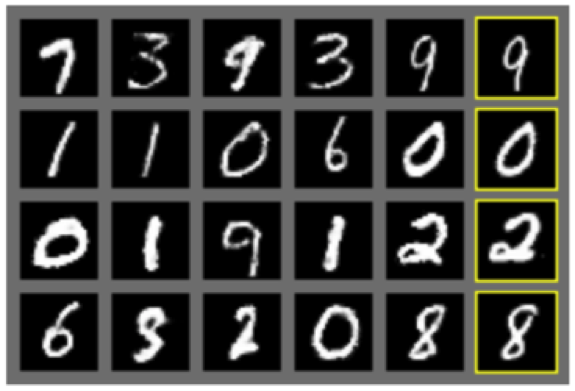Results from Goodfellow et. al. Rightmost column (in yellow boxes) are the closest images from the training dataset to the image on its direct left. All other images are generated samples.

Using a more sophisticated architecture for G and D with strided convolutional, adam optimizer instead of stochastic gradient descent, and a number of other improvements in architecture, hyperparameters and optimizers (see paper for details), we get the following results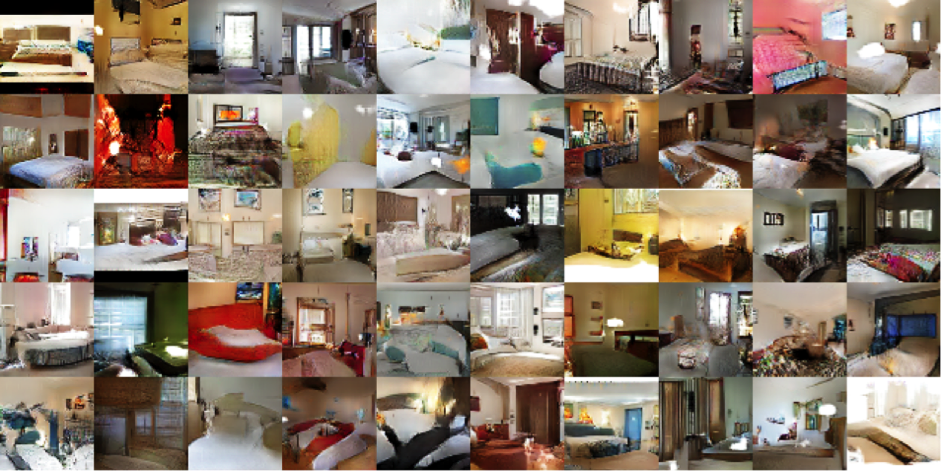Results from Alec Radford et. al. Images are of ‘bedrooms’.

By subscribing you accept KDnuggets Privacy Policy

By subscribing you accept KDnuggets Privacy Policy

By subscribing you accept KDnuggets Privacy Policy